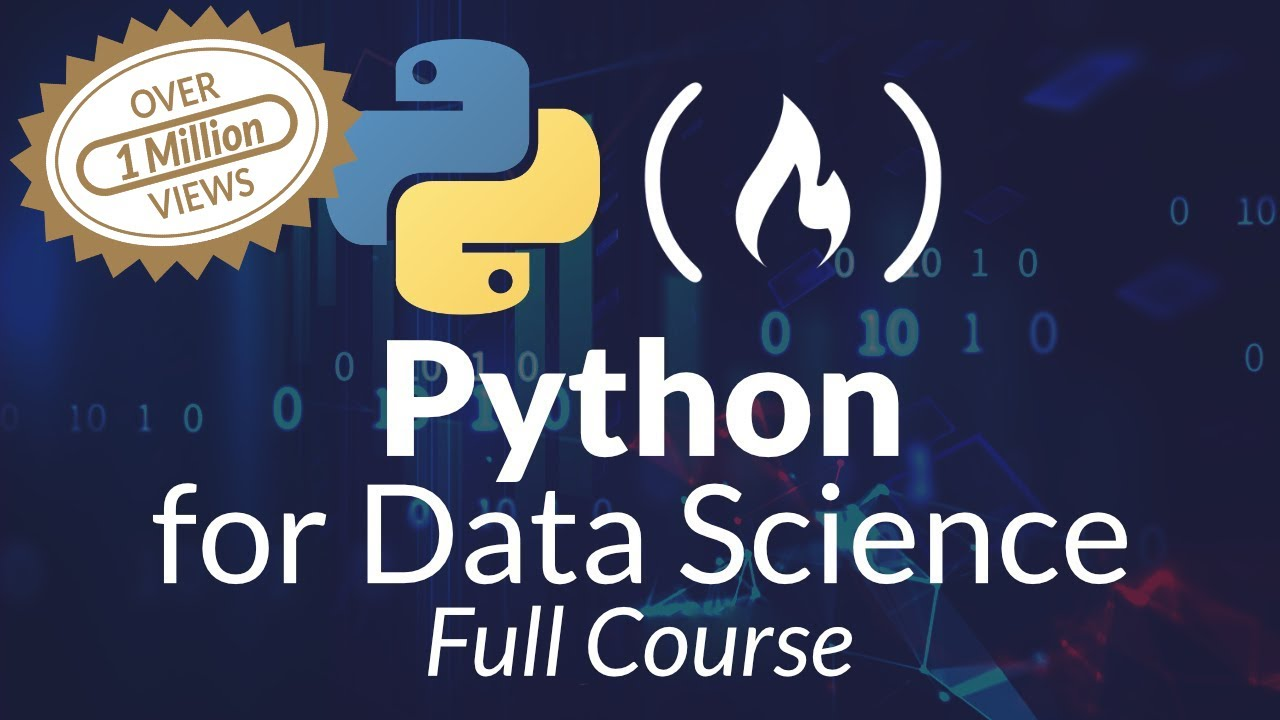# Python for Data Science - Learn Python, Pandas, NumPy, MatplotlibPython tutorial for Data Science - Learn Python, Pandas, NumPy, Matplotlib, will take you from knowing nothing about Python to coding and analyzing data with Python using tools like Pandas, NumPy, and matplotlib. This is a hands-on course and you will practice everything you learn step-by-step.

This Python data science course will take you from knowing nothing about Python to coding and analyzing data with Python using tools like Pandas, NumPy, and matplotlib.

This is a hands-on course and you will practice everything you learn step-by-step.

⭐️ Course Contents ⭐️ ⌨️ (0:00:00) Introduction to the Course and Outline ⌨️ (0:03:53) Basics of Programming ⌨️ (1:11:35) Why Python ⌨️ (1:33:09) Installation of Anaconda and Python ⌨️ (1:37:25) Launching Jupyter Notebook ⌨️ (1:46:28) Coding on the IPython Shell ⌨️ (1:53:33) Variable and Operator in Python ⌨️ (2:27:45) Boolean and Comparison in Python ⌨️ (2:55:37) Python Useful functions ⌨️ (3:20:04) Control Flow in Python ⌨️ (5:11:52) Function in Python ⌨️ (6:41:47) Module in Python ⌨️ (7:30:04) String in Python ⌨️ (8:23:57) Data Structure (List, Tuple, Set, Dictionary) in Python ⌨️ (9:36:10) Data Science Python Libraries - NumPy ⌨️ (11:04:12) Data Science Python Libraries - Pandas ⌨️ (12:01:31) Data Science Python Libraries - Matplotlib ⌨️ (12:09:00) Data Analysis with Python Libraries -COVID19 Trend Analysis

## Python Data Science – A Free 12-Hour Course for Beginners. Learn Pandas, NumPy, Matplotlib

This free 12-hour Python Data Science course will take you from knowing nothing about Python to being able to analyze data. You'll learn basic Python, along with powerful tools like Pandas, NumPy, and Matplotlib. This is a hands-on course and you will practice everything you learn step-by-step. This course includes a full codebase for your reference [https://github.com/datapublishings/Course-python-data-science]. It kicks off with a one-hour introduction to basic programming concepts, proble

## Python Pandas Tutorial | Data Science For Beginners With Python Pandas

Welcome to this course on Data Science For Beginners With Python Pandas. Learn how Perform a Many of data operations in Python's popular Pandas library.

## Basic Data Types in Python | Python Web Development For Beginners

In the programming world, Data types play an important role. Each Variable is stored in different data types and responsible for various functions. Python had two different objects, and They are mutable and immutable objects.

## Beginner’s Guide to Data Analysis using numpy and pandas

Beginner’s Guide to Data Analysis using numpy and pandas. Oftentimes, we tend to forget that the pandas library is built on top of the numpy package.

## Data Visualization With Python: Matplotlib

Data visualization is the graphical representation of data in a graph, chart or other visual formats. It shows relationships of the data with images.